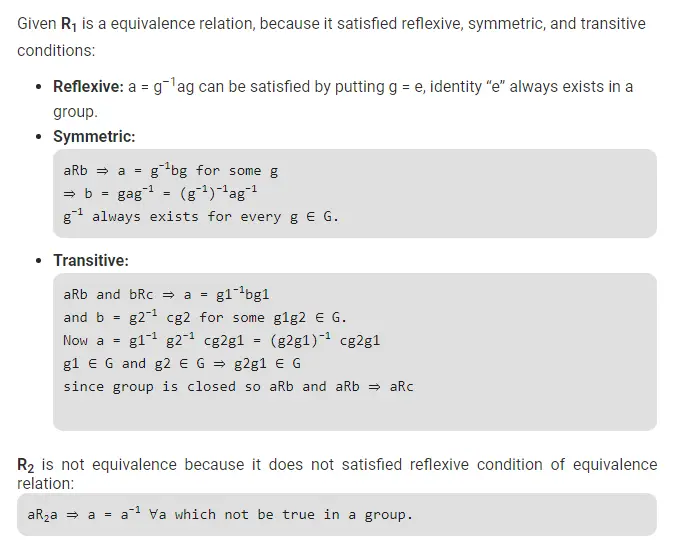# Gate CS-2019 Question Paper With Solutions

Q. 17 Let G be an arbitrary group. Consider the following relations on G:

• R1: ∀a, b ∈ G, aR1b if and only if ∃g ∈ G such that a = g−1bg
• R2: ∀a, b ∈ G, aR2b if and only if a = b−1

Which of the above is/are equivalence relation/relations?

(A) R1 and R2

(B) R1 only

(C) R2 only

(D) Neither R1 nor R2